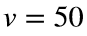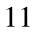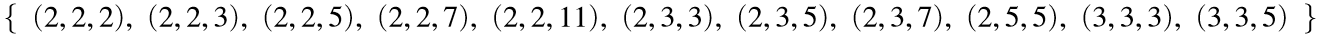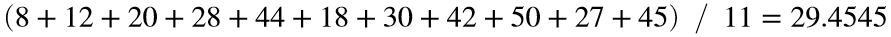# Problem 52985. Easy Sequences 47: Boxes with Prime Edges

This is related to problem "Easy Sequences 41: Boxes with Integer Edges". However, here we will be investigating a smaller-sized set, namely, the set of rectangular-faced boxes with edges representable by (not necessarily distinct) prime numbers.
Given a volume limit, v, we are asked to find the average volume of all boxes with prime edges, whose volumes are less than or equal to v.
For example forcubic units, allpossible prime-edged boxes are given below:.
Their average volume is:cubic units.

### Solution Stats

62.5% Correct | 37.5% Incorrect
Last Solution submitted on Mar 19, 2023

### Community Treasure Hunt

Find the treasures in MATLAB Central and discover how the community can help you!

Start Hunting!